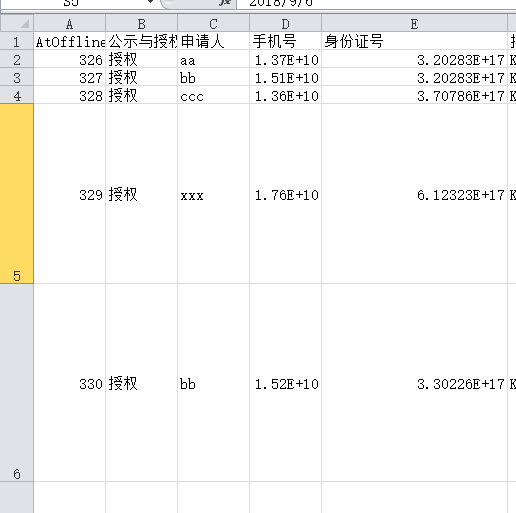vb.net 导出Excel后，格式要固定行的高度及数字转文字-线上等

1、行的高度，乎大乎小，我想统一成，比如14像素，要怎么写呢

2、手机号及身分证号是文字，但说excel就变数字了Private Sub ExcelExport_New(ByVal dt As DataTable, ByVal ProgressBar1 As Object)
ProgressBar1.Visible = True
ProgressBar1.Value = 10
'建立Excel
Dim xlApp, xlBook, xlSheet As Object
xlApp = CreateObject("Excel.Application")
xlSheet = xlBook.Worksheets(1)

``````    Dim row As DataRow                               '定义row为datatable的行
Dim col As DataColumn                            '定义col为datatable的列
Dim rowindex, colindex As Integer

'打开sheet1那页
xlSheet = xlApp.Worksheets("sheet1")

'赋初值
rowindex = 1                                        '行(原值1)
colindex = 0                                             '列
'写入字段名
For Each col In dt.Columns
colindex = colindex + 1
xlApp.Cells(1, colindex) = col.ColumnName            '原值是1，
Next

'向表格中写入具体内容
For Each row In dt.Rows
rowindex = rowindex + 1
colindex = 0
For Each col In dt.Columns
colindex = colindex + 1
xlApp.Cells(rowindex, colindex) = row(col.ColumnName)
Next
ProgressBar1.Value = rowindex * (ProgressBar1.Maximum / dt.Rows.Count - 1)
Next
'显示Excel应用程序
ProgressBar1.Value = 0
ProgressBar1.Visible = False
xlApp.Visible = True

End Sub
``````

1个回答